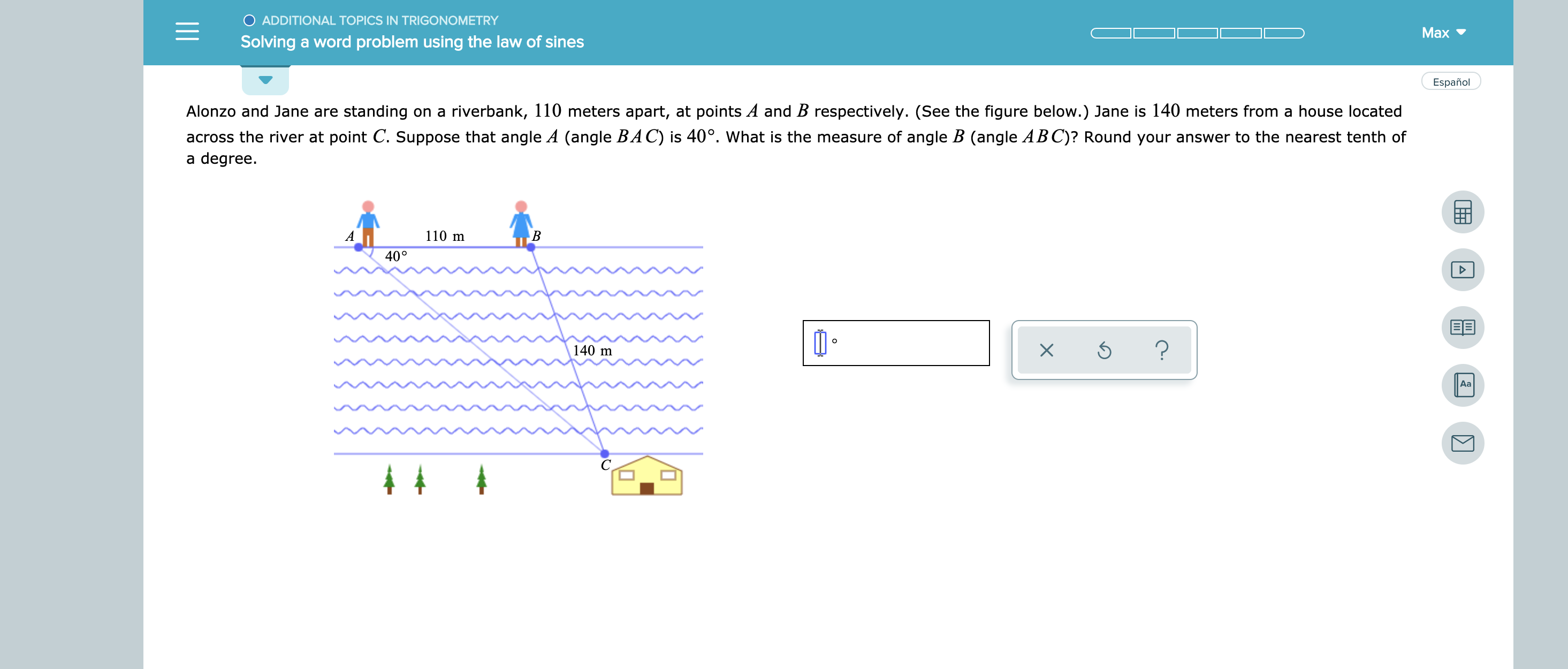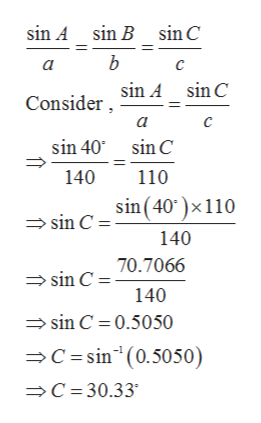# O ADDITIONAL TOPICS IN TRIGONOMETRYMaxSolving a word problem using the law of sinesEspañolAlonzo and Jane are standing on a riverbank, 110 meters apart, at points A and B respectively. (See the figure below.) Jane is 140 meters from a house locatedacross the river at point C. Suppose that angle A (angle BAC) is 40°. What is the measure of angle B (angle ABC)? Round your answer to the nearest tenth ofa degree.110 mAВ40°O?140 mАа4

Question
2 views

see attachmenthelp_outlineImage TranscriptioncloseO ADDITIONAL TOPICS IN TRIGONOMETRY Max Solving a word problem using the law of sines Español Alonzo and Jane are standing on a riverbank, 110 meters apart, at points A and B respectively. (See the figure below.) Jane is 140 meters from a house located across the river at point C. Suppose that angle A (angle BAC) is 40°. What is the measure of angle B (angle ABC)? Round your answer to the nearest tenth of a degree. 110 m A В 40° O ? 140 m Аа 4 fullscreen
check_circle

Step 1

Using the law of si...help_outlineImage Transcriptionclosesin A sin B sin C b Consider Sin A _ sinC c sin 40 sin C 140 110 sin C Sin(40)x 110 140 sin C0.7066 140 sin C 0.5050 C =sin (0.5050) C 30.33 fullscreen

### Want to see the full answer?

See Solution

#### Want to see this answer and more?

Solutions are written by subject experts who are available 24/7. Questions are typically answered within 1 hour.*

See Solution
*Response times may vary by subject and question.
Tagged in

### Other